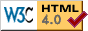Modern Portfolio Theory Applet
"> ">
The following keys and mouse actions do interesting things:
(NOTE: you may have to click on the applet once before it will accept keyboard input)
```    Left-click - move an asset (white dot)
Middle-click (or ctrl-click or alt-click) - add an asset
Right-click (or meta-click) - delete nearest asset

'c' - toggle control panel
'g' - toggle geom mean grid lines (may be very subtle depending on your monitor)
'a' - toggle arithmetic mean grid lines
'm' - toggle minor grid lines (when showing major grid lines)
'G' - switch to using geometric mean returns (default)
'A' - switch to using arithmetic mean returns
'M' - toggle showing correlation matrix in control panel

Debugging (stuff you don't care about):
' ' - repaint
'V' - cycle event debug level (output to java console window).
'T' - toggle draw time debugging (output to java console window).
'b' - toggle double buffering
```
So, I was reading this book called The Intelligent Asset Allocator by William Bernstein, and it has some fascinating pictures showing how, if you diversify your portfolio among statistically uncorrelated assets with various risk/return characteristics, you can get a portfolio with a lower risk and higher return than the lowest-risk component asset, and sometimes a higher return than even the highest-return component asset. Cool! In particular, if two assets have exactly the same risk/return characteristics, if they are statistically uncorrelated, you get much lower risk and slightly higher return (!) by mixing them 50/50 than by investing in either one by itself.

What hooked me is that it's easy to quantify and calculate the resulting return and risk, if you define risk as standard deviation. The simplest model of an asset with any given mean return and standard deviation is a coin toss. For example, consider an investment that, in any given year, has 50% chance of giving you +30% return, and 50% chance of giving you -10% return (this is Bernstein's "Uncle Fred's coin toss"). The (geometric) mean return is 8.17% (square root of (1.30 * .90)), and the standard deviation is 20%. If you have two identical but uncorrelated investments A and B with the above behavior, and you split your money 50/50 between them, then there are four equally likely outcomes in any given year:

```                  A    B
-10% -10%
-10%  30%
30% -10%
30%  30%
```
So the expected return of this portfolio is the geometric mean of these four outcomes, i.e.
```            (.90 * 1.10 * 1.10 * 1.30)1/4 = 1.0908,
```
i.e. 9.08% (slightly better than the 8.17% you'd get by putting all your money one one or the other coin toss). And the standard deviation is clearly lower than that of a single coin toss; it's approximately:
```                sqrt(((-10-10)2 + (10-10)2 + (10-10)2 + (30-10)2) / 4) = 14.14%,
```
better than the 20% of either individual coin toss. You can do a similar easy calculation to get the mean and standard deviation of a 25/75 split of A and B, or any other combination.

This applet illustrates this phenomenon. The vertical axis is (mean) annualized return, and the horizontal axis is risk, measured as standard deviation (calculated in log space, so, for example, a standard deviation of 25% means the deviation is typically +25% or -20%). (The mean annualized return is calculated in log space too, so it's a geometric mean.) (Notice that the axis labels are not evenly spaced, since it's a logarithmic scale on both axes.)

Create new assets (white points) with the middle mouse button (or alt-left), and move them around with the left mouse button. The red curves show mixtures of two assets, in increments of 5%. The blue grids show mixtures of three assets. The green dots show mixtures of four assets. The yellow dots show mixtures of five assets (very slow).
You probably don't want to display that many, since it gets too cluttered and slow; anyway, according to Bernstein,

"In the real world of investing, however, it is difficult to find two assets that are uncorrelated, and it is practically impossible to find three. It is absolutely impossible to find more than three mutually uncorrelated assets."

The upper-left edge of the data (where return is maximized for a given risk, and risk is minimized for a given return) is called the efficient frontier, and is shown delimited by two cyan dots (the minimimum-risk portfolio and the maximum-return portfolio). Finding the efficient frontier is called Mean-Variance Optimization. A guy named Harry Markowitz came up with this stuff and earned a Nobel prize for it.

William Bernstein's web site is http://www.efficientfrontier.com/ . It includes an on-line version of the aforementioned book through Chapter 2 (unfortunately the interesting stuff begins in chapter 3).

Download source code for this applet (compressed jar file contains class files and source)

 Last Modified: Sat Jul 5 23:08:17 PDT 2003 Don Hatch hatch@plunk.org